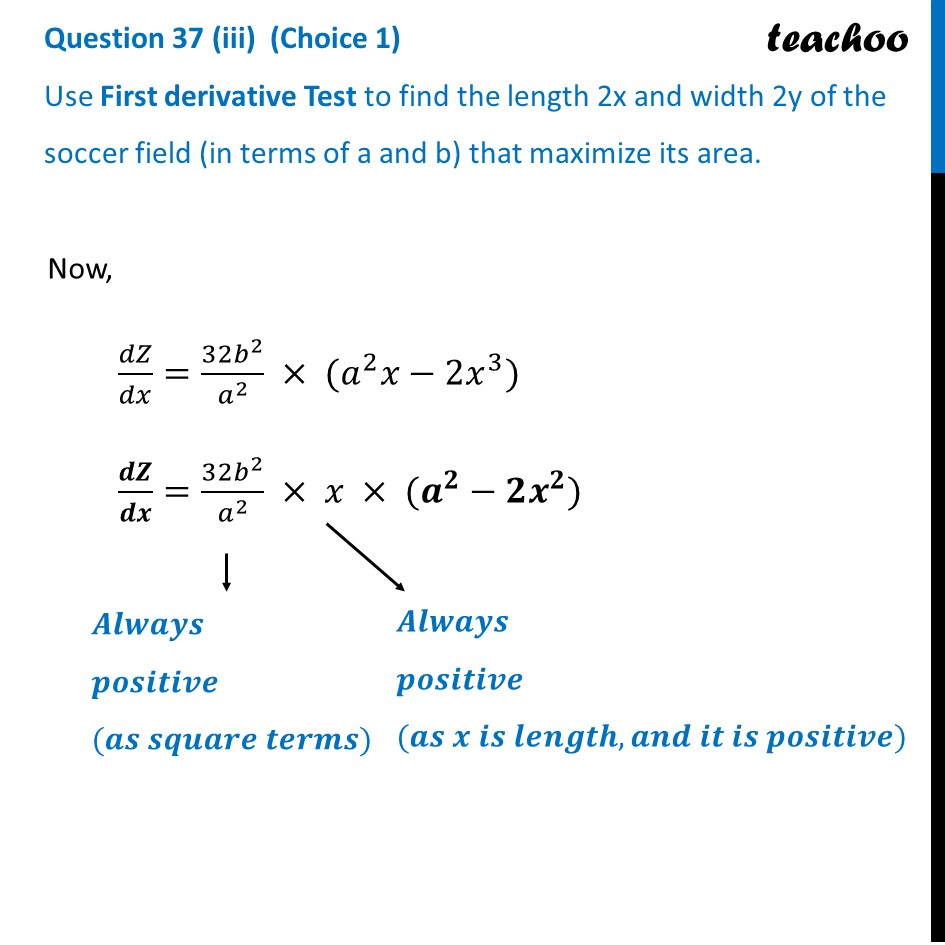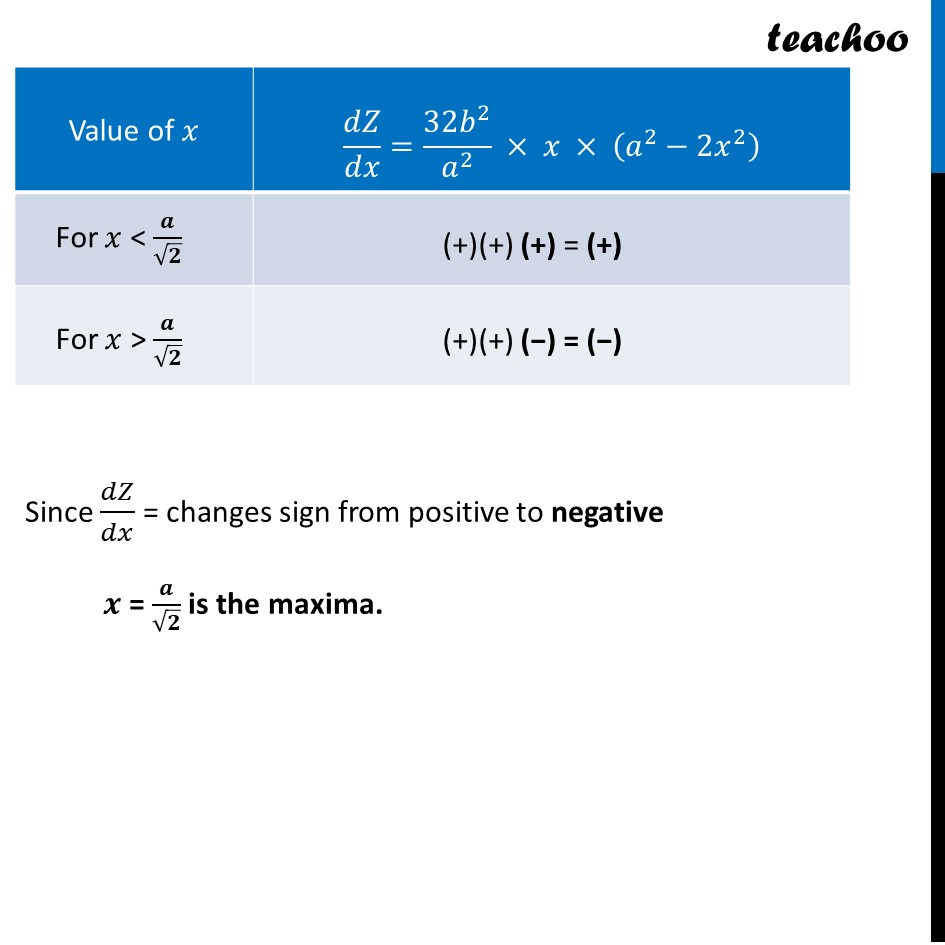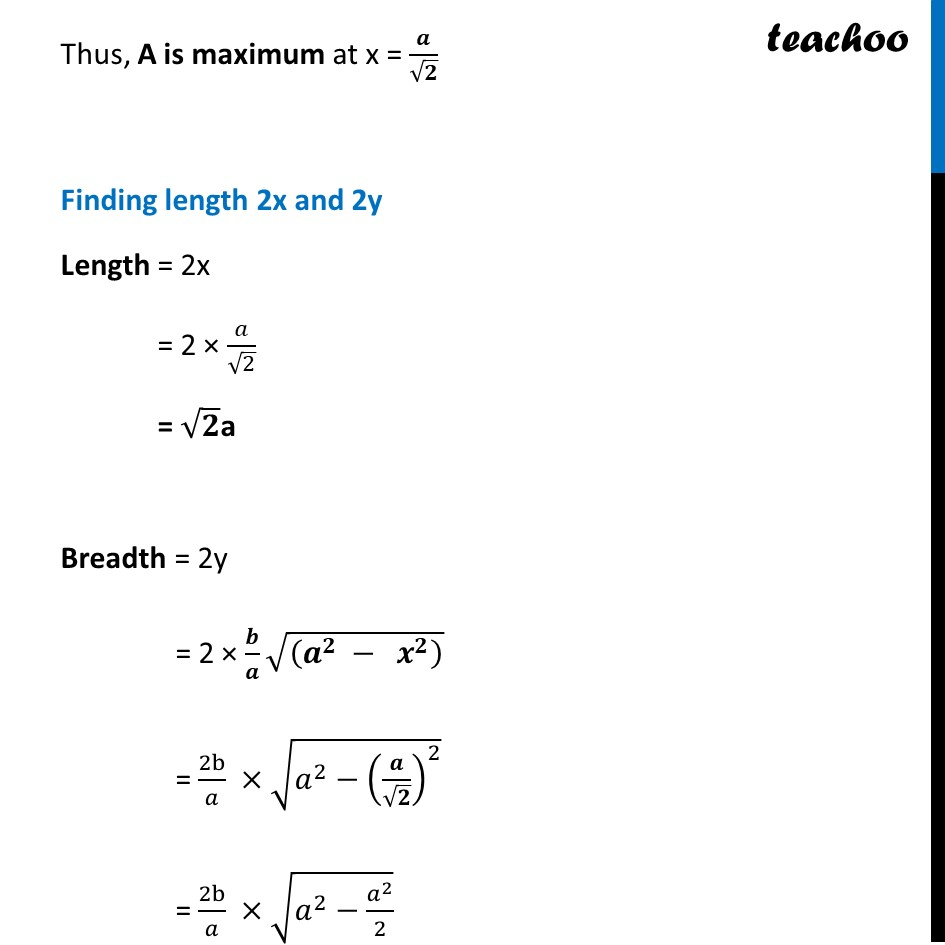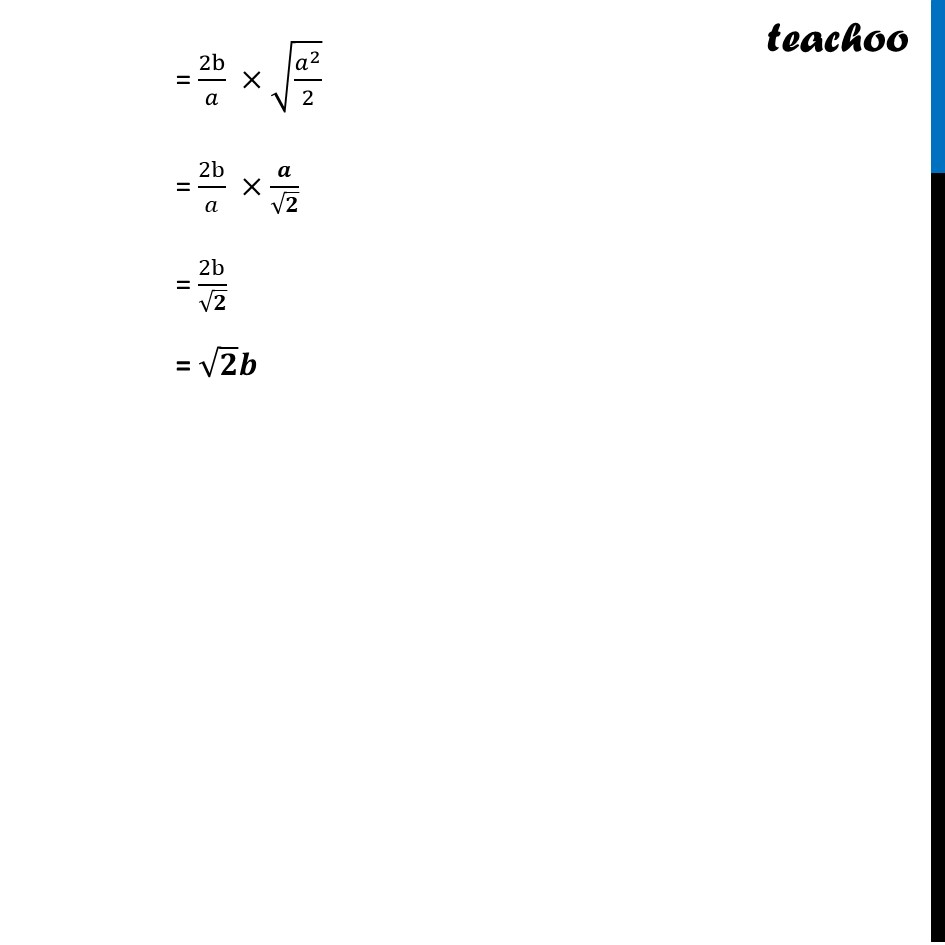CBSE Class 12 Sample Paper for 2023 Boards

Class 12
Solutions of Sample Papers and Past Year Papers - for Class 12 Boards

## Use First derivative Test to find the length 2x and width 2y of the soccer field (in terms of a and b) that maximize its area.Learn in your speed, with individual attention - Teachoo Maths 1-on-1 Class

### Transcript

Question 37 (iii) (Choice 1) Use First derivative Test to find the length 2x and width 2y of the soccer field (in terms of a and b) that maximize its area.Now, 𝑑𝑍/𝑑𝑥=(32𝑏^2)/𝑎^2 × (𝑎^2 𝑥−2𝑥^3) 𝒅𝒁/𝒅𝒙=(32𝑏^2)/𝑎^2 × 𝑥 × (𝒂^𝟐−𝟐𝒙^𝟐) Since 𝑑𝑍/𝑑𝑥 = changes sign from positive to negative 𝒙 = 𝒂/√𝟐 is the maxima. Thus, A is maximum at x = 𝒂/√𝟐 Finding length 2x and 2y Length = 2x = 2 × 𝑎/√2 = √𝟐a Breadth = 2y = 2 × (" " 𝒃)/𝒂 √((𝒂^𝟐 − 𝒙^𝟐 ) ) = 2b/𝑎 ×√(𝑎^2−(𝒂/√𝟐)^2 ) = 2b/𝑎 ×√(𝑎^2−𝑎^2/2) = 2b/𝑎 ×√(𝑎^2/2) = 2b/𝑎 ×𝒂/√𝟐 = 2b/√𝟐 = √𝟐 𝒃